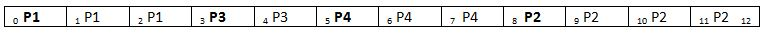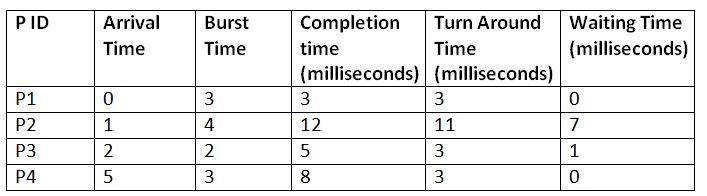# SJF: Shortest Job First Scheduling Algorithm

Submitted by Monika Sharma, on June 22, 2019

The Shortest Job Scheduling Algorithm keeps track of the Burst time of all the available processes and then assigns the processor to that process which has the shortest burst time. This is also a type of non-preemptive scheduling algorithm where once a process starts its execution, it cannot be interrupted in between its processing and any other process can be executed only after the assigned process has completed its processing and has been terminated.

The SJF scheduling algorithm is better than FCFS as the problems like the convoy effect (if a process is very large, the processes which arrive after that process has to wait for a much longer time even if they have a job of only a few time units)does not occur in this type of schedule. However, the longer processes may be delayed for a much longer period of time in this type of schedule which is its major drawback.

Let us take an example to understand it better. Suppose there are four processes with process ID's P1, P2, P3, and P4 and they enter into the CPU as follows:

Process IDArrival Time
(milliseconds)
Burst Time
(milliseconds)
P103
P214
P322
P453

So, if the OS follows the SJF algorithm for scheduling these processes, then they will be executed in the following manner:```    Total Turn Around Time = 3 + 11 + 3 + 3
= 20 milliseconds
Average Turn Around Time= Total Turn Around Time / Total No. of Processes
= 20 / 4
= 5 milliseconds

Total Waiting Time = 0 + 7 + 1 + 0
= 8 milliseconds
Average Waiting Time = Total Waiting Time / Total No. of Processes
= 8 / 4
= 2 milliseconds
```